nextnano.com  GUI: nextnanomat  Tool: nextnano++  Tool: nextnano³  Tool: nextnano.QCL  Download | Search | Copyright | News | Publications  * password protected nextnano³ software1D QW k.p dispersion

# nextnano3 - Tutorial

## Energy dispersion of holes in a quantum well

Authors: Stefan Birner, Michael Povolotskyi

If you want to obtain the input files that are used within this tutorial, please contact stefan.birner@nextnano.de.
```-> a) 1Dwell_GaAs_AlAs_nn3.in  / *_nnp.in      - ```input file for the nextnano3 and nextnano++ software``` -> b) 1Dwell_GaSb_AlSb_nn3.in  / *_nnp.in      - ```input file for the nextnano3 and nextnano++ software``` -> c) 1Dwell_InGaAs_InP_nn3.in / *_nnp.in      - ```input file for the nextnano3 and nextnano++ software``` -> d) 1DIn20Ga80AsQW_75nm_sg.in       1DIn20Ga80AsQW_75nm_kp.in       1DIn20Ga80AsQW_75nm_kp_dispersion.in  ```

## a) Unstrained GaAs/AlAs quantum well

• This tutorial is based on Fig. 1 in

Boundary conditions in multiband k.p models: A tight-binding test
S. De Franceschi, J.-M. Jancu, F. Beltram
Physical Review B 59 (15), 9691 (1999)

• This input file simulates a GaAs/AlAs structure:``` 1Dwell_GaAs_AlAs_nn3.in```
AlAs (barrier)
GaAs (well) - The well is 17 molecular layers thick (4.8 nm), located between 20 and 24.8 nm.

The picture shows the valence band edges of the quantum well structure together with three quantized states. The heavy and light hole band edges are degenerate. The red band is the split-off hole band edge. Note that these artificial band edges correspond to the bulk band edges.
Also shown are the probability densities of the three uppermost subbands (eigenfunction²). Note that each eigenstate is twofold spin-degenerate at k||=0. These eigenfunctions are plotted as positions on the energy scale that correspond to their eigenenergies, i.e. psi2 + eigenvalue (eV).
The energy scale is shifted by -1.45967 eV to refer to the bulk valence band edge of the quantum well material, i.e. the GaAs valence band edge (hh, lh) is at 0 eV.• We use a 6-band k.p model for the holes.

``` \$quantum-model-holes  model-name                     = 6x6kp       ! ```six-band k.p theory```  valence-band-numbers           = 1 2 3       ! ```heavy hole, light hole, split-off hole```  number-of-eigenvalues-per-band = 10 10 10    ! ```For each band, 10 eigenvalues should be calculated.```  quantization-along-axes        = 0 0 1  boundary-condition-001         = Dirichlet  k-range-determination-method   = k-max-input  k-range                        = 0.1d0       ! [1/Angstrom]  num-kp-parallel                = 1849        ! ```1849 = 43 * 43 = [(2 * 21) + 1 ] * [(2 * 21) + 1 ]` ==> ` 231 k|| points have to be calculated`  ... \$end_quantum-model-holes`

• k.p output:```  \$output-kp-data  destination-directory = Schroedinger_kp/  k-par-dispersion      = yes  k-par-disp-ev-min     = 1  k-par-disp-ev-max     = 10! k-par-dispersion      = yes        ! ```whole k|| space```   k-par-dispersion      = 01-00-11   ! [kx,ky]```:  from```  ```to`  `where` kx = 0 `and```                                      !         ```from```  ```to`  `where``` kx = ky   ... \$end_output-kp-data```

• ``` \$numeric-control  simulation-dimension           = 1  zero-potential                 = yes  kp-vv-term-symmetrization      = no   ! ```nonsymmetrized k.p Hamiltonian```! kp-vv-term-symmetrization      = yes  !   ```  symmetrized k.p Hamiltonian```  schroedinger-kp-discretization = box-integration  schroedinger-kp-ev-solv        = ARPACK  schroedinger-kp-residual       = 1.0d-12  schroedinger-kp-iterations     = 1200 \$end_numeric-control```

• Database: We used the Luttinger parameters (gamma1, gamma2, gamma3) given in S. De Franceschi, J.-M. Jancu, F. Beltram, Phys. Rev. B 59 (15), 9691 (1999) and also their valence band offset (0.5 eV).
The conversion from Luttinger parameters to Dresselhaus parameters (L, M, N) is described here.
For details on the bandoffset see here.
So the changes to the``` database_nn3.in ```file are as follows:

AlAs
```!Luttinger-parameters  =   3.88d0   0.69d0    1.46d0  ! [Franceschi] ```gamma1, gamma2, gamma3``` !                          0.12d0   0.03d0            ! kappa, q  6x6kp-parameters      =  -7.640d0 -3.500d0  -8.760d0 ! ```L,M,N` [hbar²/2m](--> `divide by` hbar²/2m)`
```                           0.28d0                     ! [DEFAULT] deltasplit-off ```in``` [eV]  valence-band-energies =   0.866333333333333333d0     ! [eV]```

GaAs
```!Luttinger-parameters  =   7.05d0   2.0d0     3.0d0   ! [Franceschi] ```gamma1, gamma2, gamma3``` !                          1.72d0   0.04d0            ! kappa, q  6x6kp-parameters      = -16.050d0 -4.050d0 -18.000d0 ! ```L,M,N` [hbar²/2m](--> `divide by` hbar²/2m)`
```                           0.341d0                    ! [DEFAULT] deltasplit-off ```in``` [eV]  valence-band-energies =   1.346d0                    ! [DEFAULT] [eV]```

The valence band offset is 0.5 eV (S. De Franceschi, PRB 59, 9691 (1999)) and calculated as follows:
``` ( valence-band-energies(GaAs) + deltasplit-off(GaAs)/3 ) -  ( valence-band-energies(AlAs) + deltasplit-off(AlAs)/3 ) =  ( 1.346 + 0.341/3 ) - (0.86633 + 0.28/3) = 0.5 eV``` But rather than modifying the default database, it is much better to modify the input file (`1Dwell_GaAs_AlAs.in`):
``` !---------------------------------------------------------!! ```Here we are overwriting the database entries for AlAs.                     ```       !!---------------------------------------------------------! \$binary-zb-default                                        ! binary-type               = AlAs-zb-default              ! apply-to-material-numbers = 1                            ! Luttinger-parameters      =   3.88d0   0.69d0    1.46d0  ! [Franceschi] ```gamma1, gamma2, gamma3```                                0.0d0    0.0d0             ! kappa, q  6x6kp-parameters          =  -7.640d0 -3.500d0  -8.760d0 ! ```L,M,N` [hbar²/2m](--> `divide by` hbar²/2m)`
```                               0.28d0                     ! [DEFAULT] deltasplit-off ```in``` [eV]  valence-band-energies     =   0.866333333333333333d0     ! [eV]```
``` band-shift                =  -1.459666666666666667d0     ! ==> ```hh(GaAs) = 0 eV, to adjust band alignments: adds to all band energies``` \$end_binary-zb-default                                    !!---------------------------------------------------------! !---------------------------------------------------------!! ```Here we are overwriting the database entries for GaAs.                   ```       !!---------------------------------------------------------! \$binary-zb-default                                        ! binary-type               = GaAs-zb-default              ! apply-to-material-numbers = 2                            ! Luttinger-parameters      =   7.05d0   2.0d0     3.0d0   ! [Franceschi] ```gamma1, gamma2, gamma3```                                0.0d0    0.0d0             ! kappa, q  6x6kp-parameters          = -16.050d0 -4.050d0 -18.000d0 ! ```L,M,N` [hbar²/2m](--> `divide by` hbar²/2m)`
```                               0.341d0                    ! [DEFAULT] deltasplit-off ```in``` [eV]  valence-band-energies     =   1.346d0                    ! [DEFAULT] [eV]```
``` band-shift                =  -1.459666666666666667d0     ! ==> ```hh(GaAs) = 0 eV, to adjust band alignments: adds to all band energies``` \$end_binary-zb-default                                    !!---------------------------------------------------------!  ```
• k|| dispersion for the three uppermost subbands:

The eigenvalues are twofold degenerate due to spin (and because the quantum well is symmetric). Thus eigenvalue 1 and 2 correspond to the first picture, 3 and 4 to the second one and 5 and 6 to the third one.
For the following three pictures, the energy is refered to the bulk valence band edge of the quantum well material, i.e. hh/lh(GaAs) = 0 eV. The colors and the color bar correspond to the energy given in eV.
The x and y coordinate axes refer to the in-plane wave vector. The units are in 1/Angstrom.

Subband 1 (eigenvalue 1 and 2):
```- kpar1D_disp_hl_6x6kp_qc001_ev001_2Dplot.fld /.coord /.dat  ```(1st eigenvalue)```- kpar1D_disp_hl_6x6kp_qc001_ev002_2Dplot.fld /.coord /.dat  ```(2nd eigenvalue)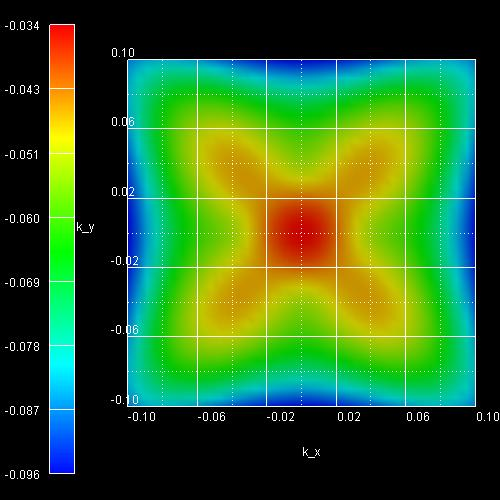Subband 2 (eigenvalue 3 and 4):```- kpar1D_disp_hl_6x6kp_qc001_ev003_2Dplot.fld /.coord /.dat  ```(3rd eigenvalue)```- kpar1D_disp_hl_6x6kp_qc001_ev004_2Dplot.fld /.coord /.dat  ```(4th eigenvalue)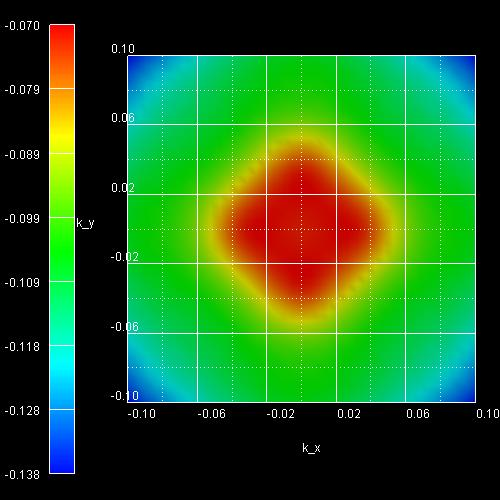Subband 3 (eigenvalue 5 and 6):
```- kpar1D_disp_hl_6x6kp_qc001_ev005_2Dplot.fld /.coord /.dat  ```(5th eigenvalue)```- kpar1D_disp_hl_6x6kp_qc001_ev006_2Dplot.fld /.coord /.dat  ```(6th eigenvalue)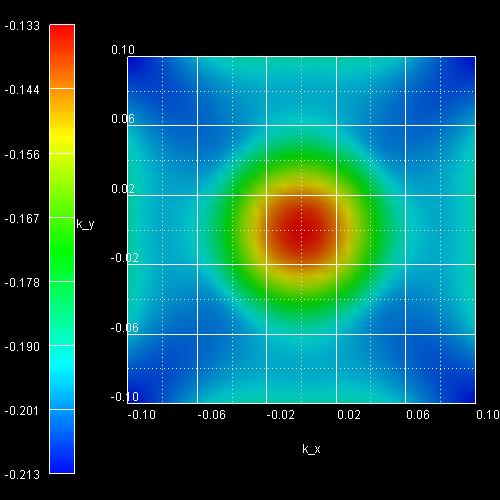• Here we plot a cut through the above three pictures from  to the zone center and from the zone center to .
This plot was obtained by plotting the following file:
```- kpar1D_disp_01_00_11_hl_6x6kp_ev_min001_ev_max010.dat  ->  abscissa, eigenvalue_i ```The value of the abscissa is found as follows:
- From  to zero we just take` -kx`.
- From zero to  we take` SQRT(kx²+ky²)`.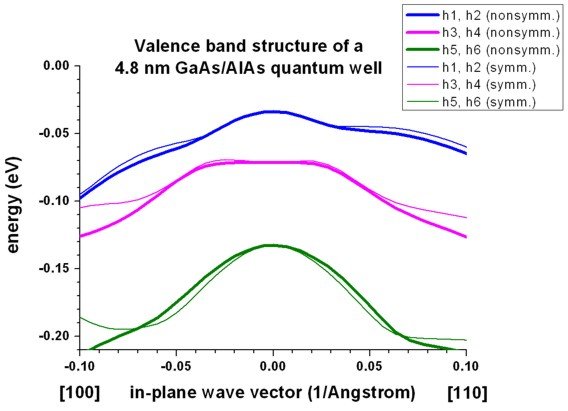The above figure shows the eigenvalues as a function of k|| vector. The three lines correspond to the upper three eigenvalues (which are two-fold spin-degenerate) as shown in the above QW figure.
The thick lines are for the nonsymmetrized k.p Hamiltonian (which is closer to the more accurate tight-binding results),
the thin lines are for the symmetrized k.p Hamiltonian.
The two sets of k.p subbands coincide at the Brillouin-zone center (i.e. at k||=0). They do not show pronounced discrepancies at nonzero in-plane k vectors. This follows from the rather small difference between the effective-mass parameters of GaAs and AlAs.
Obviously, for larger k values, the discrepancies are more significant.

• Symmetrized vs. nonsymmetrized k.p Hamiltonian

To switch between the k.p dispersion for the nonsymmetrized and symmetrized k.p Hamiltonian as in Franceschi's paper one has to change the following option:

``` \$numeric-control  simulation-dimension           = 1  kp-vv-term-symmetrization      = no   ! ```nonsymmetrized k.p Hamiltonian```! kp-vv-term-symmetrization      = yes  !   ```  symmetrized k.p Hamiltonian` `

## b) Tensilely strained GaSb/AlSb quantum well

```=> 1Dwell_GaSb_AlSb_nn3.in ```If you want to obtain the input files that are used within this tutorial, please contact stefan.birner@nextnano.de.

The following figure reproduces Fig. 2 of Franceschi's paper very well.

It is a tensiley strained 5.1 nm GaSb quantum well embedded between unstrained AlSb barriers.

The biaxial strain is 0.65 % and breaks the degeneracy of the bulk heavy and light hole band edge. Now the light hole band edge lies above the heavy hole band edge.

The figure shows that the first two subbands are nearly degenerate at the Brillouin zone center and show strong coupling.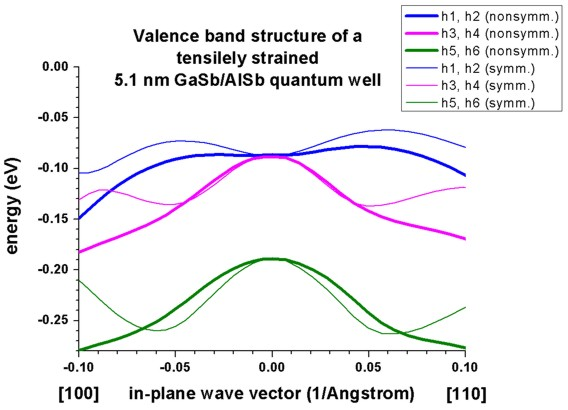A large discrepancy between the nonsymmetrized and the symmetrized k.p Hamiltonian can be seen. (See also the discussion in Franceschi's paper and their tight-binding results.)

## c) Tensilely strained In0.43Ga0.57As/InP quantum well

```=> 1Dwell_InGaAs_InP_nn3.in ```If you want to obtain the input files that are used within this tutorial, please contact stefan.birner@nextnano.de.

The following figure reproduces Fig. 3 of Franceschi's paper very well.

It is a tensiley strained 5.7 nm In0.43Ga0.57As quantum well embedded between unstrained InP barriers.

The biaxial strain is 0.73 % and breaks the degeneracy of the bulk heavy and light hole band edge. Now the light hole band edge lies above the heavy hole band edge.Again, a large discrepancy between the nonsymmetrized and the symmetrized k.p Hamiltonian can be seen. (See also the discussion in Franceschi's paper and their tight-binding results.)

## d) Strained In0.2Ga0.8As/GaAs quantum well

```=> 1DIn20Ga80AsQW_75nm_sg.in    1DIn20Ga80AsQW_75nm_kp.in    1DIn20Ga80AsQW_75nm_kp_dispersion.in ```If you want to obtain the input files that are used within this tutorial, please contact stefan.birner@nextnano.de.

These input files have been used for Fig. 8 in the following paper:

Dimensionally constrained D’yakonov–Perel’ spin relaxation in n-InGaAs channels: transition from 2D to 1D
A.W. Holleitner, V. Sih, R.C. Myers, A.C. Gossard, D.D. Awschalom
New Journal of Physics 9, 342 (2007)

• ```=> 1DIn20Ga80AsQW_75nm_sg.in ```A 7.5 nm In0.2Ga0.8As quantum well is sandwiched between two GaAs layers. The quantum well is grown pseudomorphically on a GaAs substrate and is thus strained compressively with respect to the GaAs substrate.

The GaAs is n-type doped with Si with a concentration of 3 x 1017 cm-3 in the regions between 50 and 80 nm and between 127.5 and 137.5 nm.

Consequently, we first have to solve the single-band Schrödinger equation together with the Poisson equation self-consistently, in order to obtain the electrostatic potential. The electron ground state is below the Fermi level.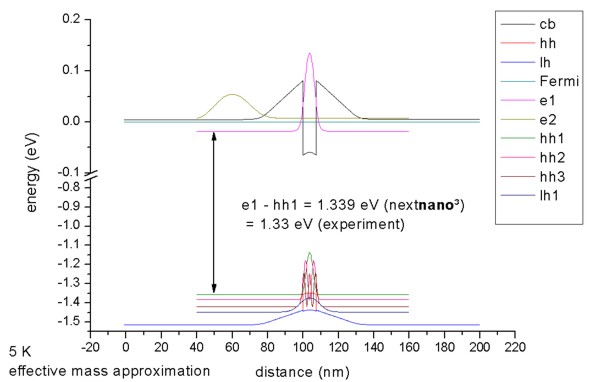• ```=> 1DIn20Ga80AsQW_75nm_kp.in ```
The calculated electrostatic potential is read in and then the 8-band k.p equation is solved to get the eigenstates for k||=0.
The calculated transition energy between the ground state electron and the ground state (heavy) hole is 1.340 eV. (Note: The exciton correction has not been considered and is of the order 4 meV.)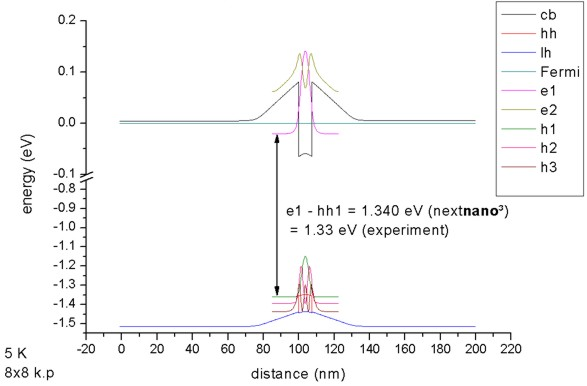For k||=0, the three highest hole states have heavy hole character whereas the forth state has light hole character. No further states are confined. The split-off hole band edge is far away from the heavy and light hole band edges (~ 0.3 eV).• `=> 1DIn20Ga80AsQW_75nm_kp_dispersion.in`

We read in the electrostatic potential again and calculate the 8-band k.p dispersion for k||/=0. This time the calculation is more time-consuming as the Schrödinger equation has to be solved for 250 different k|| points, i.e. the CPU time is 250 times larger than for k||=0 only.

For | k|| |` <= `0.02 1/Angstrom, the directions  and  are practically identical for the uppermost hole level.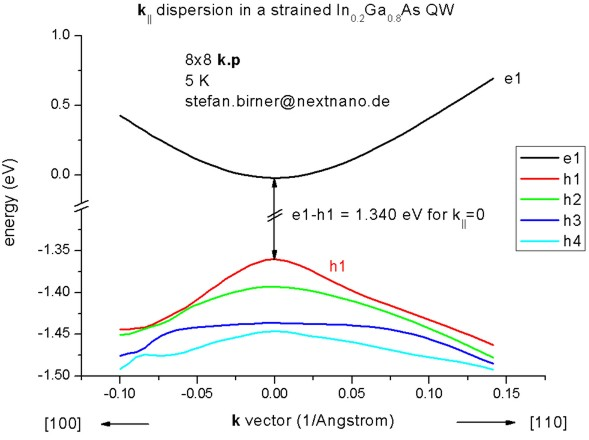• The following figure shows the k|| dispersion of the highest hole state (h1).
The x axis shows the kx value between `-`0.10 [1/Angstrom] and 0.10 [1/Angstrom], the y axis shows ky.
The maximum energy of the hole state occurs at -1.3603 eV at (kx,ky) = (0,0), i.e. in the center of the figure (Gamma point).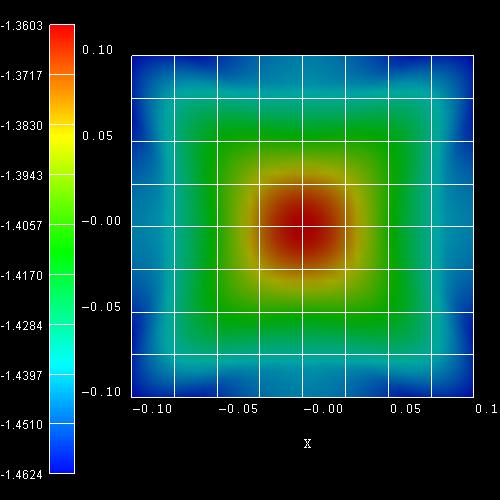• Please help us to improve our tutorial! Send comments to``` support [at] nextnano.com```.